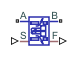# Spool Orifice Flow Force (IL)

Axial fluid force on spool orifice in an isothermal liquid system

Since R2020a

•Libraries:
Simscape / Fluids / Valve Actuators & Forces

## Description

The Spool Orifice Flow Force (IL) block models the hydraulic axial force on a spool orifice. It receives the spool position as a physical signal at port S. You can also model the flow through a spool orifice with round or rectangular holes. A positive force acts to close the orifice.

If you would like to model the spool and axial force in one block, use the Spool Orifice (IL) block. The axial force is output as a physical signal at port F.

### Flow Force

The force on the spool is calculated as:

`$F=\frac{-{\stackrel{˙}{m}}_{A}^{2}}{\rho A}\mathrm{cos}\left(\alpha \right)\epsilon ,$`

where:

• ${\stackrel{˙}{m}}_{A}$ is the mass flow rate at port A.

• ρ is the fluid density.

• A is the orifice open area, which is determined by the spool position and orifice parameterization.

• α is the jet angle, which is calculated from an approximation of the Von Mises formula:

`${\alpha }_{jet}=0.3663+0.8373\left(1-{e}^{\frac{-h}{1.848c}}\right),$`

where c is the Radial clearance and h is the orifice opening.

• ε is the opening orientation, which indicates orifice opening that is associated with a positive or negative signal at S.

### Opening Area

The orifice opening is based on the open area created by the displaced spool:

`$\Delta S=\left(S-{S}_{\mathrm{min}}\right)\epsilon ,$`

where:

• Smin is the Spool position at closed orifice.

• S is the displacement signal at port S.

If Δs falls below 0, the orifice leakage area is used. If ΔS is greater than the Spool travel between closed and open orifice, the maximum orifice area is used.

Round Holes

Setting Orifice geometry to ```Round holes``` evenly distributes a user-defined number of holes along the sleeve perimeter that have equal diameters and centers aligned in the same plane.

The open area is calculated as:

`${A}_{orifice}={n}_{0}\frac{{d}_{0}^{2}}{8}\left(\theta -\mathrm{sin}\left(\frac{\theta }{2}\right)\right)+{A}_{leak},$`

where:

• n0 is the number of holes.

• d0 is the diameter of the holes.

• θ is the orifice opening angle:

`$\theta ={\mathrm{cos}}^{-1}\left(1-2\frac{\Delta S}{{d}_{0}}\right).$`

If θ is greater than , θ remains at .

• Aleak is the Leakage area.

The maximum open area is:

`${A}_{\mathrm{max}}=\frac{\pi }{4}{d}_{0}^{2}{n}_{0}+{A}_{leak}.$`

Rectangular Slot

Setting Orifice geometry to ```Rectangular slot``` models one rectangular slot in the tube sleeve.

For an orifice with a slot in a rectangular sleeve, the open area is

`${A}_{orifice}=w\Delta S+{A}_{leak},$`

where w is the orifice width.

The maximum opening distance between the sleeve and case is:

`${A}_{\mathrm{max}}=w\Delta {S}_{\mathrm{max}}+{A}_{leak}.$`

where ΔSmax is the Spool travel between closed and open orifice.

### Numerically-Smoothed Displacement

At the extremes of the orifice opening range, you can maintain numerical robustness in your simulation by adjusting the block . The block applies a smoothing function to every calculated displacement, but primarily influences the simulation at the extremes of this range.

If the parameter is nonzero, the block smoothly saturates the orifice opening between `0` and ΔSmax where ΔSmax is the:

• Value of the Diameter of round holes parameter, when Orifice parameterization is set to `Round holes`.

• Value of the Spool travel between closed and open orifice parameter, when Orifice parameterization is set to ```Rectangular slot```.

## Ports

### Conserving

expand all

Liquid entry or exit port to the block.

Liquid entry or exit port to the block.

### Input

expand all

Spool displacement in m, specified as a physical signal.

### Output

expand all

Axial flow force in N, specified as a physical signal.

## Parameters

expand all

Whether the modeled spool has round holes or a rectangular slot in the sleeve.

Diameter of all holes on the sleeve.

#### Dependencies

To enable this parameter, set Orifice geometry to `Round holes`.

Number of holes along the sleeve circumference.

#### Dependencies

To enable this parameter, set Orifice geometry to `Round holes`.

Rectangular slot width.

#### Dependencies

To enable this parameter, set Orifice geometry to `Rectangular slot`.

Maximum orifice opening, including the Spool position at maximum orifice area.

#### Dependencies

To enable this parameter, set Orifice geometry to `Rectangular slot`.

Radial distance between the spool and the sleeve.

Initial offset if the spool is extended or retracted in its neutral position.

Direction of member movement that opens the orifice. A positive orientation indicates that positive control member displacement increases the orifice opening. A negative orientation indicates that negative control member displacement increases the orifice opening. The magnitude is always positive.

Continuous smoothing factor that introduces a layer of gradual change to the flow response when the valve is in near-open or near-closed positions. Set this value to a nonzero value less than one to increase the stability of your simulation in these regimes.

 Manring, N. Hydraulic Control Systems. John Wiley & Sons, 2005.

 Merritt, H. Hydraulic Control Systems. Wiley, 1967.